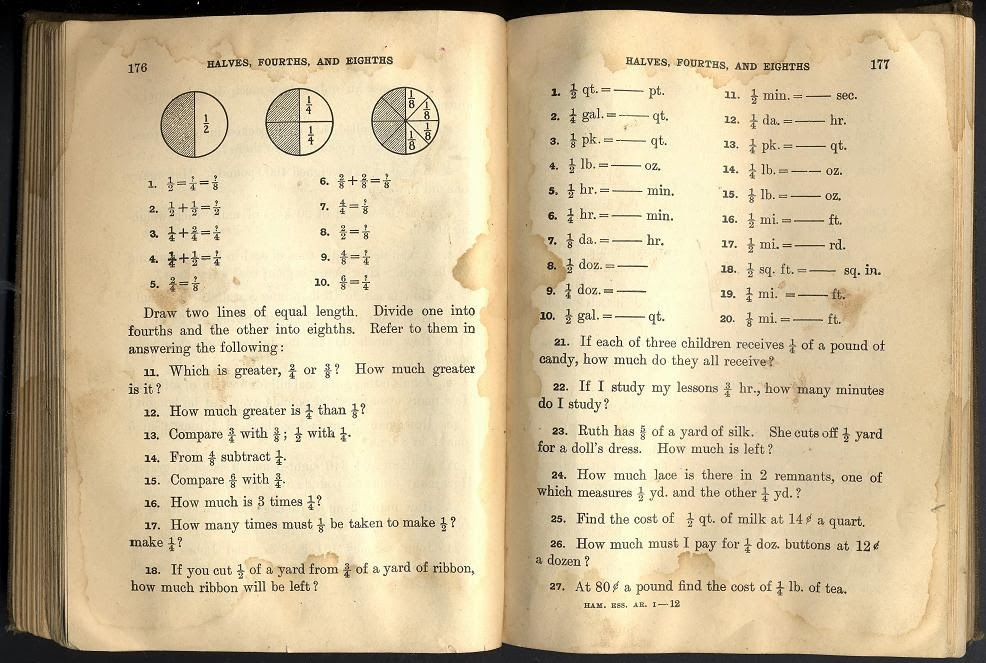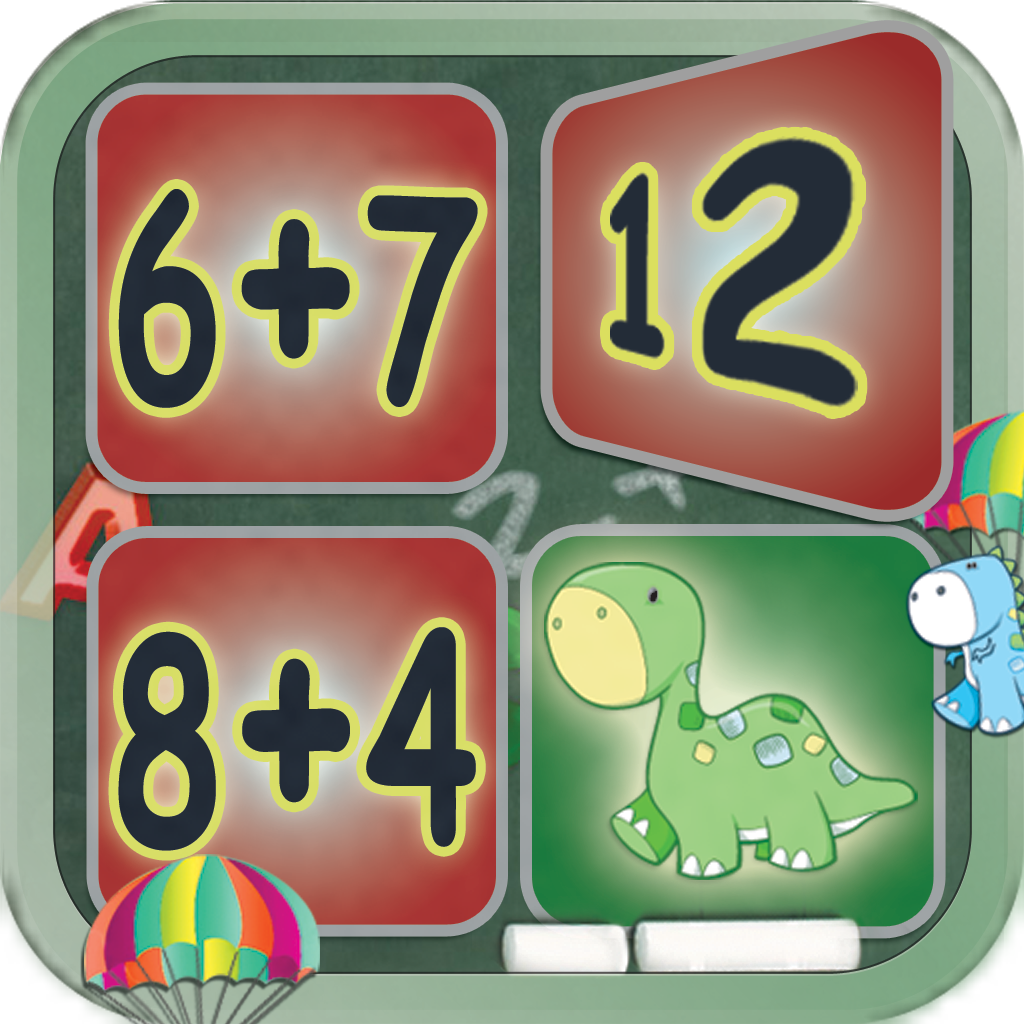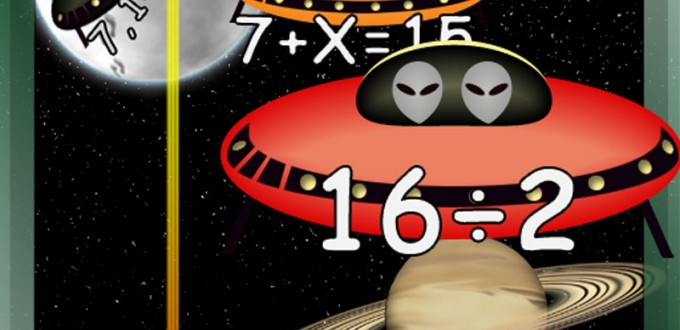@Reks: Educational iOS Applications

2nd Grade Printable Math Quiz Workshe...You can download a number of math practice worksheets designed for Grade 2  Elementary School. The practice worksheets are based on Math Essentials textbook and math common core standards. Unit 1 covers the following concepts: add and subtract numbers up to 20 add three or four numbers find partners and totals write equations for math mountains tell […]

5th Grade Printable Math Quiz SheetsThis page offers a number of math worksheets for Grade 5 based on Math Essentials math textbook and common core standards. Unit 1 covers the following topics: – fractions – simplify fractions – addition and subtraction of fractions – word problems with fractions Tap/click the following links to print math tests from Unit 1: 1. 5th Grade Unit 1 […]

4th-Grade Printable Math QuizSheetsThis page offers a number of math worksheets for Grade 4 following Math Essentials math textbook and common core standards. The worksheets are organized in four different lessons (Units). The Math topics in the first lesson (Unit) are as follows: – Calculate area and perimeter of triangle, square, rectangle, parallelogram, or trapezoid – Order of […]

Common Core Elementary School Math St...AtReks math apps rely on on common core math standards in the U.S.A.  They are reliable follow-up resources for common core standardized lessons. AtReks math apps include math facts covering kindergarten through 3rd grade school curriculum. They are all based on the finding of the National Research Council:

Addition: Math Facts Card Matching Ga...This memory game focusing on addtion is designed to encourage higher level thinking and reasoning in a fun, and friendly atmosphere. A significant advantage of this particular memory game is that the student is expected to calculate each mathematical expression to be able to connect it to its sum. Addition: Math Facts Card Matching Game […]Play one of eight Arithmetic Invaders games to defend the solar system, learn the names  of the planets and master the Grade 1 arithmetic. * Three addition games * Two subtraction games * Two multiplication games * One division game * Basic facts about the Solar System * Focus on Math problems to avoid random […]